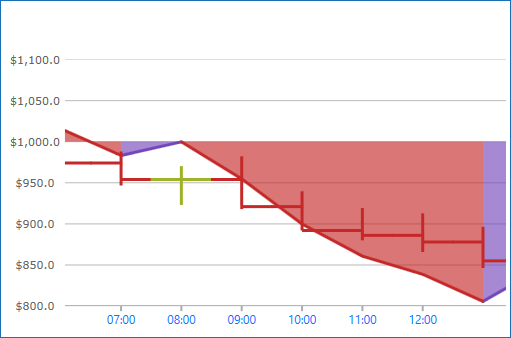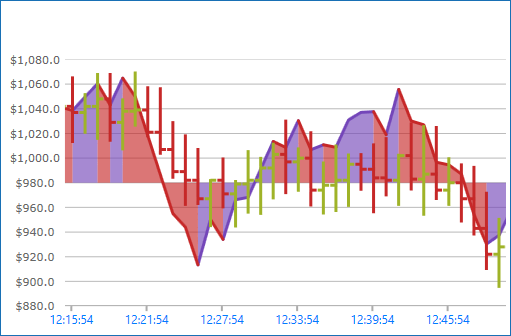Version

# Using Time X-Axis

This topic demonstrates, with code examples, how to use TimeXAxis in the XamDataChart™ control. The benefit of using TimeXAxis is to dynamically change label formats as you zoom in or out of the data. In addition, axis breaks can be created which omit dates within a range. For example, weekends can be skipped.

The topic is organized as follows:

Note: The following sections are only required if the default labels (i.e. formatting & intervals) need to be changed.

## Introduction

The TimeXAxis may be used with the following series:

For more information on what axis types are required by a specific series, refer to the Series Requirements topic.

 Note Note: All category axes require data binding and data mapping in order to show labels on the axis lines. Refer to the Getting Started with Data Chart topic for code example how to bind data to the category.Figure 1: Sample implementation of the TimeXAxis

## Axis Break Properties

The TimeXAxis has the option to exclude intervals of data with Breaks. As a result, labels will not appear at the excluded interval. For example, working/non-working, holidays, and/or weekends.

An instance of TimeAxisBreak can be added to the Breaks property and configured by using a unique Start, End and Interval.

The following code snippets show how to skip labels on the TimeXAxis in the XamDataChart for a range of years, causing uneven intervals. The result is shown in Figure 2 below.Figure 2: TimeXAxis displaying data from Monday through Friday in 2010.

In XAML:

``````<ig:XamDataChart x:Name="DataChart" >
<ig:XamDataChart.Axes>
<ig:TimeXAxis x:Name="xAxis" ItemsSource="{StaticResource data}" DateTimeMemberPath="Date" >
<ig:TimeXAxis.Breaks>
<ig:TimeAxisBreak
Start="2009-12-26T00:00:00"
End="2009-12-27T23:59:59.99"
Interval="7:00:00:00.00"/>
</ig:TimeXAxis.Breaks>
</ig:TimeXAxis>
</ig:XamDataChart.Axes>
</ig:XamDataChart>``````

In Visual Basic:

``````Dim axisBreaks = xAxis.Breaks

.Start = New DateTime(2009, 12, 26, 0, 0, 0), _
.[End] = New DateTime(2009, 12, 27, 23, 59, 59), _
.Interval = New TimeSpan(7, 0, 0, 0, 0) _
})``````

In C#:

``````var axisBreaks = xAxis.Breaks;

{
Start = new DateTime(2009, 12, 26, 00, 00, 00),
End = new DateTime(2009, 07, 12, 27, 59, 59),
Interval = new TimeSpan(7, 0, 0, 0, 0)
});``````

## Axis Label Format Properties

The TimeXAxis has the LabelFormats property which is a collection of type TimeAxisLabelFormat. Each TimeAxisLabelFormat added to the collection is responsible for assigning a unique Format and Range. This can be especially useful for drilling data from years to milliseconds and adjusting the labels depending on the range of time shown by the chart.

Name Type Description

`string`

Specifies what format to use for the visible range.

`TimeSpan`

Specifies the visible range at which the axis label formats will switch to a different format. For example, if you have two TimeAxisLabelFormat with a range set to 10 days and another set to 5 hours, as soon as the visible range in the axis becomes less than 10 days it will switch to 5 hour format.

 Note Note: The visible range is determined by the dates currently visible along the axis minus the dates that are removed by axis breaks.

The following lists a typical set of label formats for the given amount of time in view:

1. 1825 days or more (eg. 5 years) will result in a format of "yyyy".

2. 365 days or more (eg. 1 year) will result in a format of "MMM yy".

3. 1 day or more will result in a format of "MMM dd".

4. 5 hours or more will result in a format of "hh:mm".

5. Below 5 hours will result in a format of "hh:mm:ss".Figure 3: The XamDataChart control with a custom TimeAxisLabelFormat of "hh:mm:ss"

In XAML:

``````<ig:XamDataChart x:Name="DataChart" >
<ig:XamDataChart.Axes>
<ig:TimeXAxis x:Name="xAxis" ItemsSource="{StaticResource data}" DateTimeMemberPath="Date" >
<ig:TimeXAxis.LabelFormats>
<ig:TimeAxisLabelFormat
Format="hh:mm:ss"
Range="0.00:00:00"/>
<ig:TimeAxisLabelFormat
Format="hh:mm"
Range="0.05:00:00"/>
<ig:TimeAxisLabelFormat
Format="MMM dd"
Range="1.00:00:00" />
<ig:TimeAxisLabelFormat
Format="MMM yy"
Range="365.0:00:00" />
<ig:TimeAxisLabelFormat
Format="yyyy"
Range="1825.0:00:00" />
</ig:TimeXAxis.LabelFormats>
</ig:TimeXAxis>
</ig:XamDataChart.Axes>
</ig:XamDataChart>``````

In Visual Basic:

``````Dim DataChart = New XamDataChart()
Dim timeXAxis = New TimeXAxis()

Dim axisFormat = xAxis.LabelFormats
.Format = "hh:mm:ss", _
.Range = New TimeSpan(0, 0, 0, 0, 0) _
})

.Format = "hh:mm", _
.Range = New TimeSpan(0, 5, 0, 0, 0) _
})

.Format = "MMM dd", _
.Range = New TimeSpan(1, 0, 0, 0, 0) _
})

.Format = "MMM yy", _
.Range = New TimeSpan(365, 0, 0, 0, 0) _
})

.Format = "yyyy", _
.Range = New TimeSpan(1825, 0, 0, 0, 0) _
})``````

In C#:

``````var DataChart = new XamDataChart();
var timeXAxis = new TimeXAxis();

var axisFormat = xAxis.LabelFormats;

{
Format = "hh:mm:ss",
Range = new TimeSpan(0, 0, 0, 0, 0)
});

{
Format = "hh:mm",
Range = new TimeSpan(0, 5, 0, 0, 0)
});

{
Format = "MMM dd",
Range = new TimeSpan(1, 0, 0, 0, 0)
});

{
Format = "MMM yy",
Range = new TimeSpan(365, 0, 0, 0, 0)
});

{
Format = "yyyy",
Range = new TimeSpan(1825, 0, 0, 0, 0)
});``````

## Axis Intervals Properties

The TimeXAxis replaces the conventional Interval property with an Intervals collection of type TimeAxisInterval. Each TimeAxisInterval added to the collection is responsible for assigning a unique Interval, Range and IntervalType. This can be especially useful for drilling data from years to milliseconds to provide unique spacing between labels depending on the range of time shown by the chart.

Name Type Description

`double`

Specifies the interval to use. This is tied to IntervalType property. If IntervalType is set to `Days` then the numeric value specified in Interval will be in days.

`TimeSpan`

Specifies the visible range at which the axis interval will switch to a different interval. For example, if you have two TimeAxisInterval with a range set to 10 days and another set to 5 hours, as soon as the visible range in the axis becomes less than 10 days it will switch to the interval whose range is 5 hours.

Specifies the unit of time for this interval.

 Note Note: The visible range is determined by the dates currently visible along the axis minus the dates that are removed by axis breaks.

The following screenshot and code snippets demonstrate how to implement several TimeAxisInterval in the XamDataChart control.Figure 4: TimeXAxis using a TimeAxisInterval with an Interval of 12 days.

In XAML:

``````<ig:XamDataChart x:Name="DataChart" >
<ig:XamDataChart.Axes>
<ig:TimeXAxis x:Name="xAxis" ItemsSource="{StaticResource data}" DateTimeMemberPath="Date" >
<ig:TimeXAxis.Intervals>
<ig:TimeAxisInterval
Range="0.00:00:00"
Interval="36"
IntervalType="Seconds" />
<ig:TimeAxisInterval
Range="0.00:30:00"
Interval="5"
IntervalType="Minutes" />
<ig:TimeAxisInterval
Range="0.05:00:00"
Interval="1"
IntervalType="Hours" />
<ig:TimeAxisInterval
Range="1.00:00:00"
Interval="12"
IntervalType="Days" />
<ig:TimeAxisInterval
Range="365.00:00:00"
Interval="1"
IntervalType="Months" />
<ig:TimeAxisInterval
Range="1825.0:00:00"
Interval="1"
IntervalType="Years" />
</ig:TimeXAxis.Intervals>
</ig:TimeXAxis>
</ig:XamDataChart.Axes>
</ig:XamDataChart>``````

In Visual Basic:

``````Dim axisIntervals = xAxis.Intervals

.IntervalType = TimeAxisIntervalType.Seconds, _
.Interval = 36, _
.Range = New TimeSpan(0, 0, 0, 0, 0) _
})

.IntervalType = TimeAxisIntervalType.Minutes, _
.Interval = 6, _
.Range = New TimeSpan(0, 0, 30, 0, 0) _
})

.IntervalType = TimeAxisIntervalType.Hours, _
.Interval = 1, _
.Range = New TimeSpan(0, 5, 0, 0, 0) _
})

.IntervalType = TimeAxisIntervalType.Days, _
.Interval = 12, _
.Range = New TimeSpan(1, 0, 0, 0, 0) _
})

.IntervalType = TimeAxisIntervalType.Months, _
.Interval = 1, _
.Range = New TimeSpan(365, 0, 0, 0, 0) _
})

.IntervalType = TimeAxisIntervalType.Years, _
.Interval = 2, _
.Range = New TimeSpan(1825, 0, 0, 0, 0) _
})``````

In C#:

``````var axisIntervals = xAxis.Intervals;

{
IntervalType = TimeAxisIntervalType.Seconds,
Interval = 36,
Range = new TimeSpan(0, 0, 0, 0, 0)
});

{
IntervalType = TimeAxisIntervalType.Minutes,
Interval = 6,
Range = new TimeSpan(0, 0, 30, 0, 0)
});

{
IntervalType = TimeAxisIntervalType.Hours,
Interval = 1,
Range = new TimeSpan(0, 5, 0, 0, 0)
});

{
IntervalType = TimeAxisIntervalType.Days,
Interval = 12,
Range = new TimeSpan(1, 0, 0, 0, 0)
});

{
IntervalType = TimeAxisIntervalType.Months,
Interval = 1,
Range = new TimeSpan(365, 0, 0, 0, 0)
});